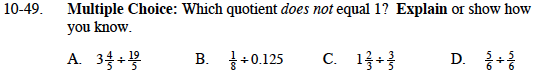### Home > MC1 > Chapter 10 > Lesson 10.1.4 > Problem10-49

10-49.If you convert each part to fractions that have the same denominator it will be easier to tell if the quotient is 1.
For example, the quotient for part (d) can be easily determined. It is, in fact, 1.

$3\frac{4}{5}=\frac{5}{5}+\frac{5}{5}+\frac{5}{5}+\frac{4}{5}=\frac{19}{5}$

$\frac{0.125}{1}=\frac{x}{8}$

$1\frac{2}{3}=\frac{3}{3}+\frac{2}{3}=\frac{5}{3}$